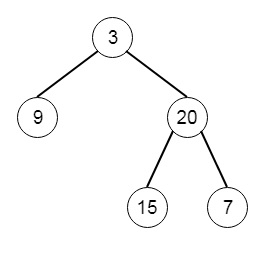# Binary Tree Preorder Traversal in Python

Suppose we have a binary tree. We have to return the preorder traversal of that tree. So if the tree is like −Then the preorder traversal will be: [3,9,20,15,7]

To solve this, we will follow these steps −

• make empty lists called res and st.
• node := root
• while node or st is not empty
• while node is not null, then
• insert val of node into res, insert node into st and set node := left of node
• temp := last element of st, and delete last element of st
• if right of temp is available, then
• node := right of temp
• return res

Let us see the following implementation to get better understanding −

## Example

Live Demo

class TreeNode:
def __init__(self, data, left = None, right = None):
self.data = data
self.left = left
self.right = right
def insert(temp,data):
que = []
que.append(temp)
while (len(que)):
temp = que
que.pop(0)
if (not temp.left):
temp.left = TreeNode(data)
break
else:
que.append(temp.left)
if (not temp.right):
temp.right = TreeNode(data)
break
else:
que.append(temp.right)
def make_tree(elements):
Tree = TreeNode(elements)
for element in elements[1:]:
insert(Tree, element)
return Tree
class Solution(object):
def preorderTraversal(self, root):
res = []
st = []
node = root
while node or st:
while node:
if node.data != None:
res.append(node.data)
st.append(node)
node = node.left
temp = st[-1]
st.pop()
if temp.right:
node = temp.right
return res
ob1 = Solution()
print(ob1.preorderTraversal(head))

## Input

[3,9,20,null,null,15,7]

## Output

[3, 9, 20, 15, 7]

Updated on: 04-May-2020

1K+ Views